Importance: High ✭✭✭
 Author(s):
 Subject: Graph Theory » Basic Graph Theory » » Cycles
 Keywords: cover cycle Eulerian
 Posted by: mdevos on: March 7th, 2007
Conjecture   Ifis a 6-edge-connected Eulerian graph andis a 2-transition system for, thenhas a compaible decomposition.

Definition: Letbe an Eulerian graph and for every vertex, letbe a partition of the edges incident with. We calla transition system. If every member ofhas size at most(for every), then we calla-transition sytem. A compatible decomposition ofis a list of edge-disjoint cycles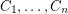with unionso that every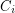contains at most one edge from every member of.

Letbe a graph and letbe the graph obtained fromby replacing each edgeof G by two edges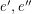in parallel. Letbe the 2-transition system ofwithwheneverand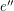are incident with. Now,is an Eulerian graph and every compatible decomposition of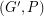gives a cycle double cover of. Since the cycle double cover conjecture can be reduced to graphs which are 3-edge-connected, the above conjecture would imply the cycle double cover conjecture.

We define a transition systemto be admissable if every member ofcontains no more than half of the edges in any edge-cut. It is easy to see that if there is a compatible decomposition of, thenmust be admissable. The converse of this is not true; There is an admissable 2-transition system of the graph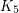which does not admit a compatible decomposition. Recently, G. Fan and C.Q. Zhang [FZ] have proved thatdoes have a compatible decomposition wheneveris admissable andhas nominor. This result imporoved upon an earlier theorem of Fleischner and Frank [FF]. Very recently, I have proved a weak version of the above conjecture, by showing thatalso has a compatible decomposition when P is a 2-transition system and G is 80-edge-connected. I'd quite like to see an improvement on this bound. Here is a related conjecture.

Conjecture  (Sabidussi)   Let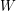be an Euler tour of the graph. Ifhas no vertex of degree two, then there is a cycle decomposition of, say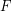, so that no two consecutive edges ofare in a common circuit of.

Ifis given bythen we may form a 2-transition systemby puttinginfor every(working modulo). Now a compatible decomposition ofis precisely a cycle decomposition ofsatisfying the above conjecture. Thus, Sabidussi's conjecture is equivalent to the assertion thathas a compatible decomposition wheneverhas no vertex of degree two andis a 2-transition system which comes from an Euler tour.

Letbe a directed Eulerian graph and for every vertex, letbe a partition of the edges incident withinto pairs so that every in-edge is paired with an out-edge. We define a compatible decomposition to be a decomposition ofinto directed circuits so that every directed circuit contains at most one edge from every member of. Our current techniques don't seem to shed any light on the problem of finding compatible decompositions for Eulerian digraphs. Next I pose a very basic question which is still open.

Problem  (DeVos)   Does there exist a fixed integersuch thathas a compatible decomposition wheneveris a-edge-connected directed Eulerian graph andis a 2-transition system?